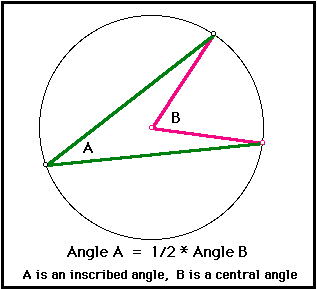MATH325: Sample Test 1

Name:

You may use pencil, pens, ruler, compass and scissors on this test. You may not use a calculator, book or notes. Please take your time on each problem and show your work in the space provided. If you get stuck on a problem, move on to the next and return when you have time.

1. What axiom or axioms guarantee that "any two points determine a straight line"? Justify your answer.
2. Shown below is a triangle ABC and a line l. Sketch the result of translating ABC three inches to the right and then reflecting it across line l.
```            A

B
C
```

```

```
3. Prove that if the diameter of a circle bisects a chord AB of a circle, and AB is not a diameter of the circle, then the diameter is perpendicular to the chord AB.
4. Using only lemmas and theorems from sections 1.1-1.5 of the text, show that if B is the center of the circle shown below, the measure of the angle at B is twice the measure of the angle at A.This practice test is similar to but harder than the actual test to be given in class. Also, the actual test will include space for you to work problems in.

Outcomes:

• Know Euclid's five axioms
• Prove theorems based on a given set of axioms, lemmas, and previously proved theorems.

Study exercises 1.15, 1.17, 1.25; be able to apply SSS, SAS, ASA; "easy" proofs like theorems 1.4.6, 1.5.1; be prepared to draw pictures illustrating isometries.
Tentative Solutions